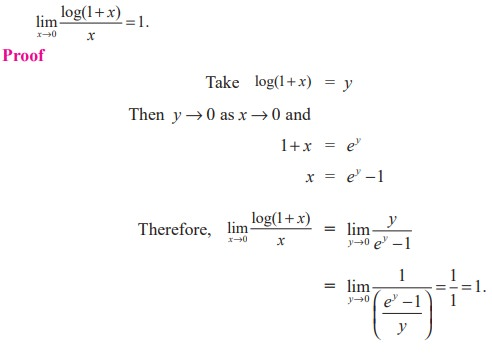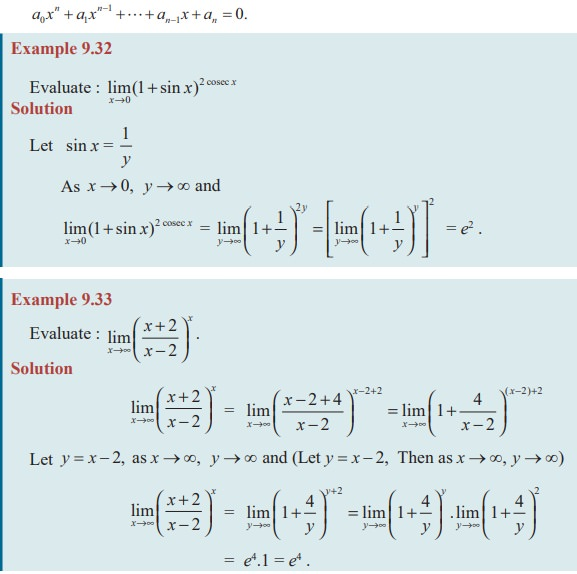Home | | Maths 11th std | Some important other limits

# Some important other limits

Differential Calculus: Limits : Some important limits

Some important other limits

Result 9.2Result 9.3Proof

We know that a x ,loga x are inverses of each other.

Since log f (x) is the inverse of  exp( f (x)), exp(log f (x)) = f (x).

Therefore, ax  = exp(loga x)Result 9.4## Some important limits without proofThis number e is also known as transcendental number in the sense that e never satisfies a polynomial (algebraic) equation of the form## EXERCISE 9.4

Evaluate the following limits :Tags : Mathematics , 11th Mathematics : UNIT 9 : Differential Calculus Limits and Continuity
Study Material, Lecturing Notes, Assignment, Reference, Wiki description explanation, brief detail
11th Mathematics : UNIT 9 : Differential Calculus Limits and Continuity : Some important other limits | Mathematics